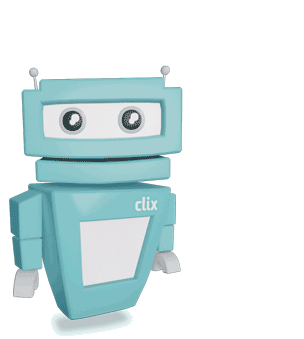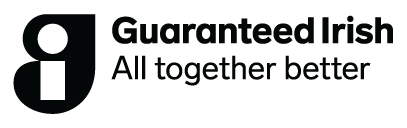Flashcards
1 of 4
Chemical Equilibrium | Definitions
Chemical Equilibrium
The state in which the rate of forward reaction is equal to the rate of reverse reaction.
Chemical Equilibrium is Dynamic
At equlibrium, the forward and reverse reactions are continuously occurring.
Le Chatlier's Principle
If a system at equilibrium is subjected to a stress, the equilibrium will shift in such a way as to minimise the stress.
Factors That Affect Equilibrium
Change in temperature, change in concentration, the addition of a catalyst (at the beginning of the reaction only) and a change in pressure (only affects gases).Deck complete
You’ve reviewed 4 terms. Keep up the good work!
tap to flip
Chemical Equilibrium | Definitions
Chemical EquilibriumThe state in which the rate of forward reaction is equal to the rate of reverse reaction.
Chemical Equilibrium is DynamicAt equlibrium, the forward and reverse reactions are continuously occurring.
Le Chatlier's PrincipleIf a system at equilibrium is subjected to a stress, the equilibrium will shift in such a way as to minimise the stress.
Factors That Affect EquilibriumChange in temperature, change in concentration, the addition of a catalyst (at the beginning of the reaction only) and a change in pressure (only affects gases).
Cut dotted horizontal lines. Fold vertical line.
Chemical Equilibrium
The state in which the rate of forward reaction is equal to the rate of reverse reaction.
Chemical Equilibrium is Dynamic
At equlibrium, the forward and reverse reactions are continuously occurring.
Le Chatlier's Principle
If a system at equilibrium is subjected to a stress, the equilibrium will shift in such a way as to minimise the stress.
Factors That Affect Equilibrium
Change in temperature, change in concentration, the addition of a catalyst (at the beginning of the reaction only) and a change in pressure (only affects gases).
Related subjects & topics
Explore similar posts in our community